# Sketch shear and moment diagrams including deflected shapes. (Indicate exact values) 2.1) PP 2PP L/2 Shear...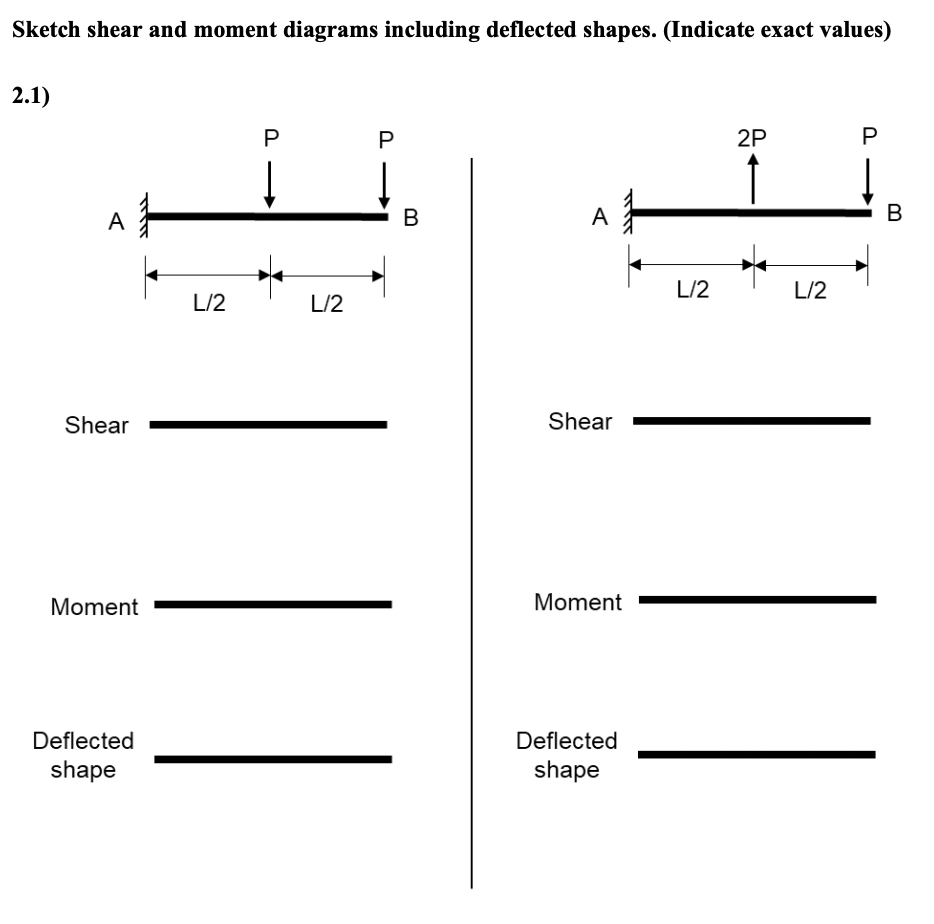Sketch shear and moment diagrams including deflected shapes. (Indicate exact values) 2.1) PP 2PP L/2 Shear Shear Moment Moment Deflected shape Deflected shape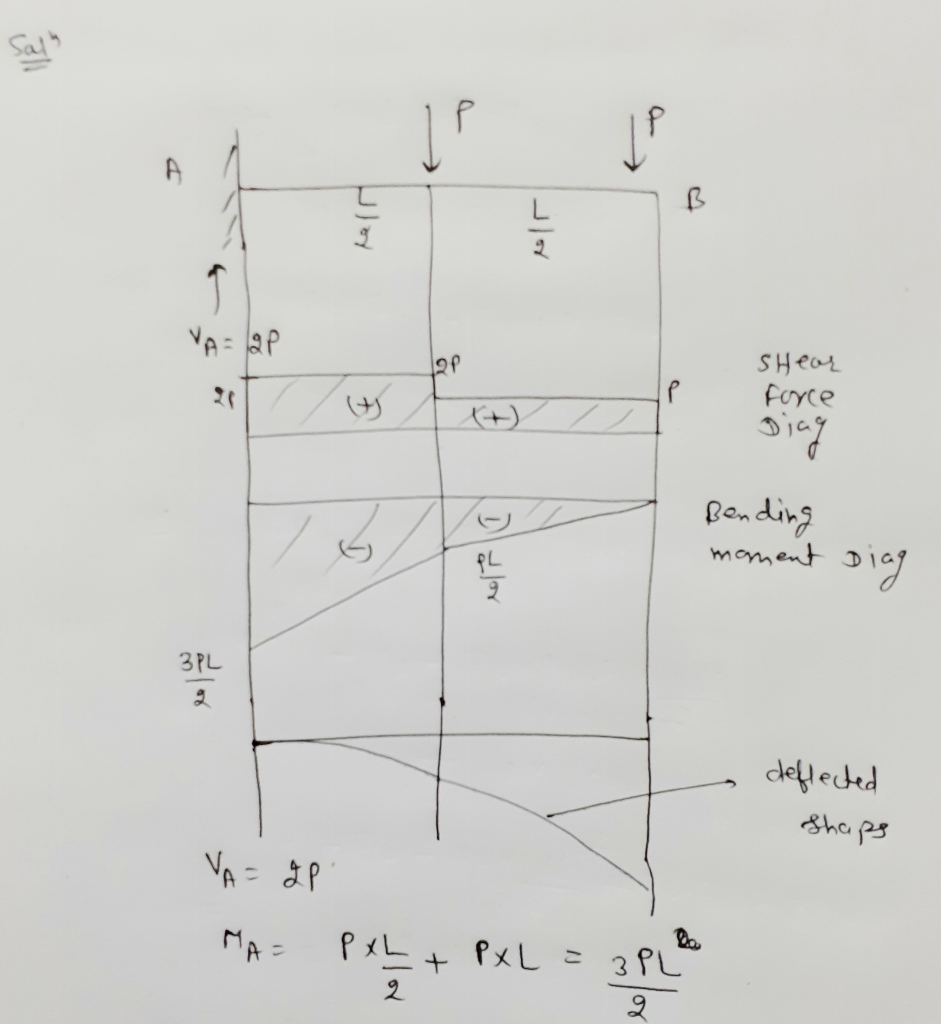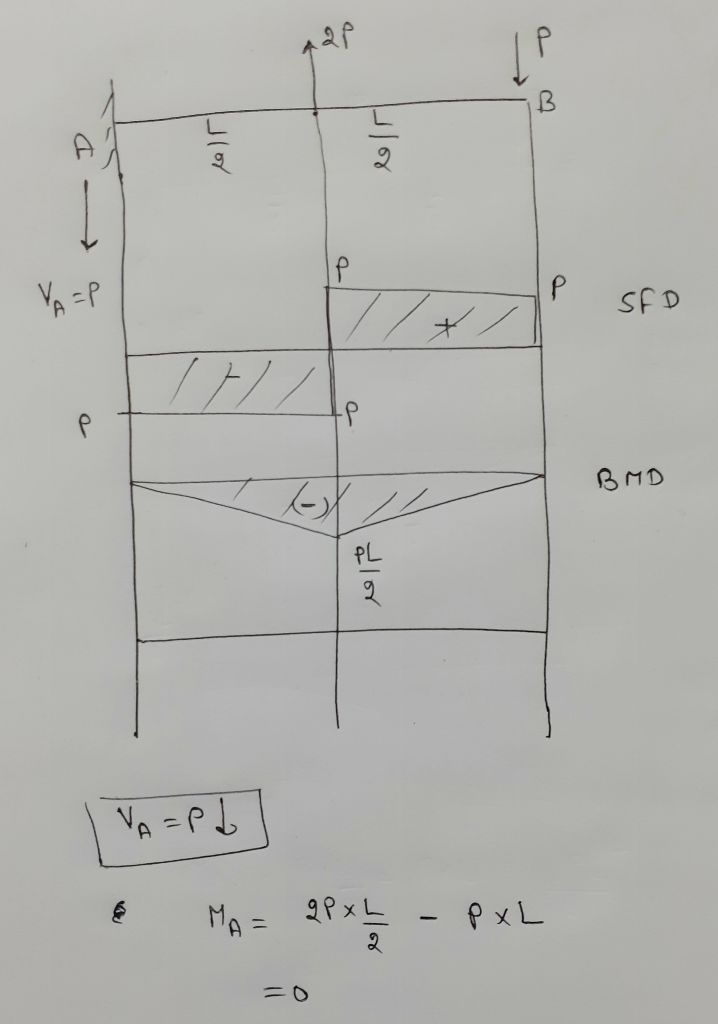#### Earn Coin

Coins can be redeemed for fabulous gifts.

Similar Homework Help Questions
• ### Draw the shear and moment diagrams including deflected shapes. 12. 3L14 Shear Shear Moment Moment Deflected...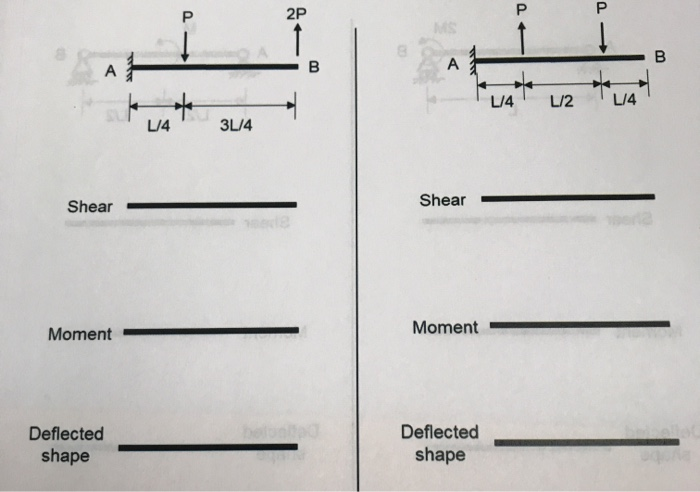Draw the shear and moment diagrams including deflected shapes. 12. 3L14 Shear Shear Moment Moment Deflected shape Deflected shape w2 12 Shear Shear Moment Moment Deflected shape Deflected shape

• ### Draw the Shear & Moment Diagrams and sketch the deflected shape for the beams shown below...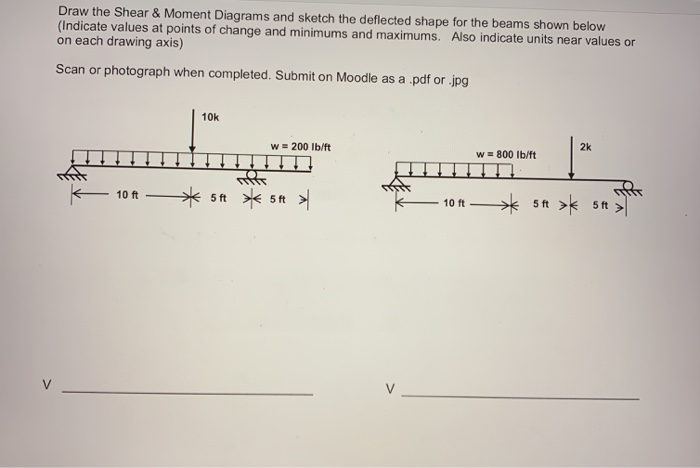Draw the Shear & Moment Diagrams and sketch the deflected shape for the beams shown below (Indicate values at points of change and minimums and maximums. Also indicate units near values or on each drawing axis) Scan or photograph when completed. Submit on Moodle as a .pdf or .jpg 10k w = 200 lb/ft 2k W = 800 lb/ft 10 ft 5 ft 5ft > 10 ft ** 5+ > 5 > M M D D

• ### a Determine the reactions b) Draw the shear and moment diagrams c) Sketch the deflected shape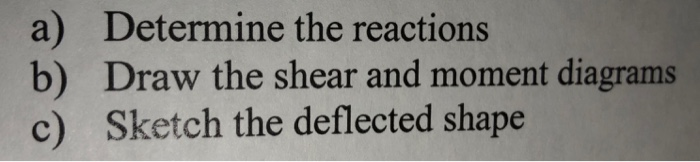a Determine the reactions b) Draw the shear and moment diagrams c) Sketch the deflected shape

• ### Analyze the Frames shown below. Draw the shear and moment diagrams and sketch the approximate deflected...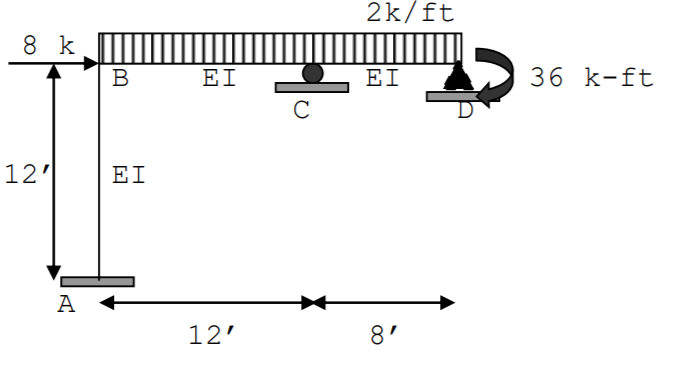Analyze the Frames shown below. Draw the shear and moment diagrams and sketch the approximate deflected shape Settlement at A = 1” and at C = .5” E = 30000 kis, I = 240 in4 2k/ft 8 k B EI EI 36 k-ft С 12'1 EI A 12' 8'

• ### For beam given below Draw the shear- and moment diagrams. Label all important values of shear...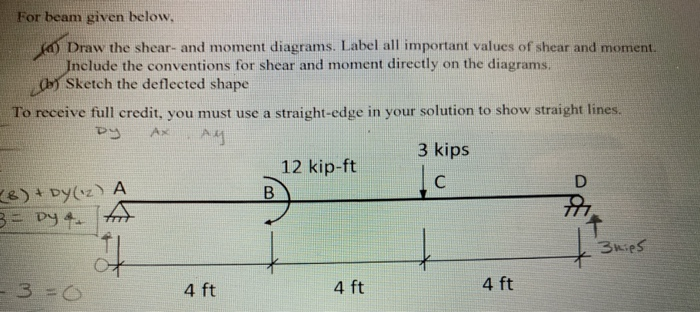For beam given below Draw the shear- and moment diagrams. Label all important values of shear and moment. nclude the conventions for shear and moment directly on the diagrams Sketch the deflected shape To reeiv leredr you mus ue astraighto sbwg ns 3 kips 12 kip-ft 4 ft 4 ft 4 ft

• ### Construct the shear and bending moment diagrams and the qualitative deflected shape for each of the...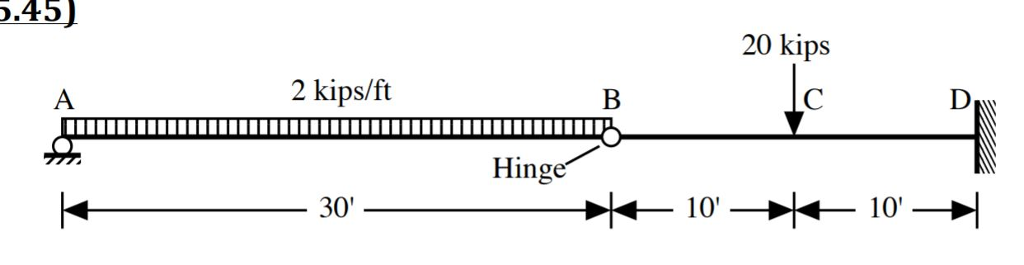Construct the shear and bending moment diagrams and the qualitative deflected shape for each of the beams shown. 5.45) 20 kips 2 kips/ft Hing 10' 10' 30

• ### do the 4th question plz 1-(25%) Draw shear and moment diagrams for the beam shown in Figure P-1. Draw a sketch of the deflected shape 2-(25%) Using moment area method, for the beam shown in Figure...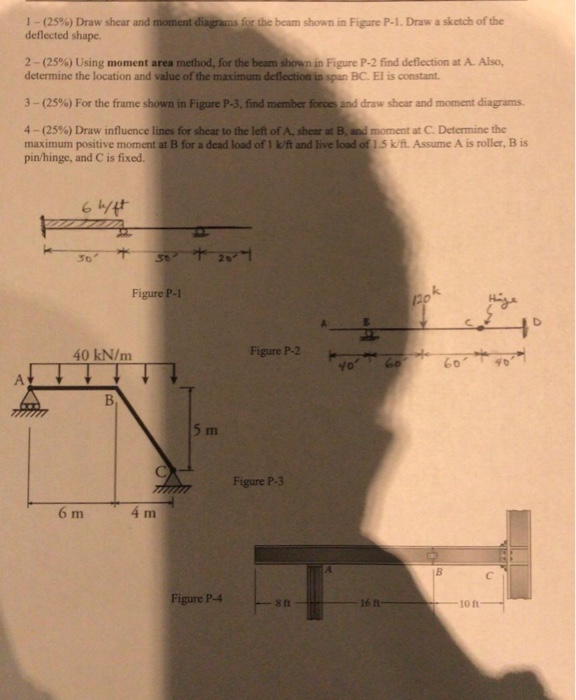do the 4th question plz 1-(25%) Draw shear and moment diagrams for the beam shown in Figure P-1. Draw a sketch of the deflected shape 2-(25%) Using moment area method, for the beam shown in Figure P-2 find deflection at A. Also, determine the location and value of the maximum deflection in span BC. El is constant 3-(25%) For the frame shown in Figure P-3-find member frees and draw shear and moment diagrams. 4-(25%) Draw/ influence lines for shear to...

• ### 3. Without writing shear and moment equations, draw shear and moment diagrams and indicate the values...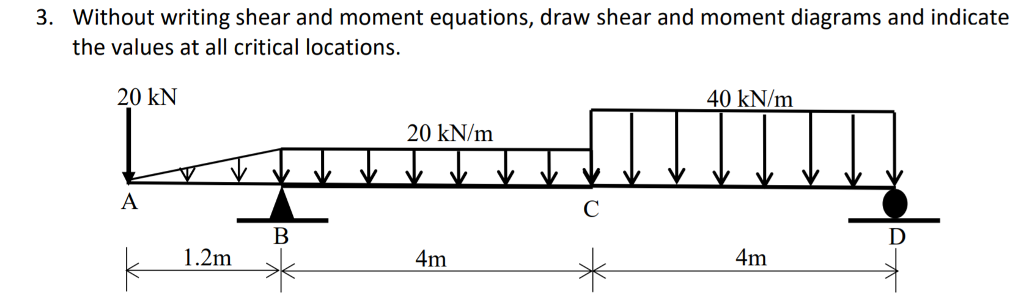3. Without writing shear and moment equations, draw shear and moment diagrams and indicate the values at all critical locations. 20 kN 40 kN/m 20 kN/m 1.2m 4m 4m

• ### 1-(25%) Draw shear and moment diagrams for the beam shown in Figure P-1 . Draw a sketch of the deflected shape. The spans are 30-ft long each (total of 90-ft), the concentrated loads are eac...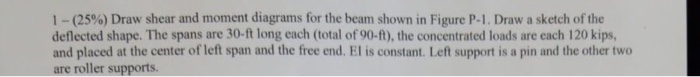1-(25%) Draw shear and moment diagrams for the beam shown in Figure P-1 . Draw a sketch of the deflected shape. The spans are 30-ft long each (total of 90-ft), the concentrated loads are each 120 kips, and placed at the center of left span and the free end. El is constant. Left support is a pin and the other two are roller supports. 120 kips 120 kips Figure P-1 1-(25%) Draw shear and moment diagrams for the beam shown...

• ### beam - shear, bending moment diagrams with graphical method

Sketch the shear diagram and the moment diagram for beamAE in Fig 5.4-25. (Sketch these on paper. Your instructormay ask to collect them.)Figure 5.4-25.Use the Graphical Method to sketch V(x) andM(x), as illustrated in Examples 5.7 through5.9. That is, use theinformation in Table 5.1 in the textin sketching the shear diagram and the moment diagram. Draw therequested shear-force and bending-moment diagrams approximatelytoscale. Label all critical ordinates, including the maximum andminimum values, and indicate the sections at which these occur.